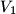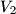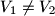IMO Shortlist 1960 problem 6

Kvaliteta:
Avg: 0,0
Težina:
Avg: 0,0
Consider a cone of revolution with an inscribed sphere tangent to the base of the cone. A cylinder is circumscribed about this sphere so that one of its bases lies in the base of the cone. let$V_1$ be the volume of the cone and$V_2$ be the volume of the cylinder.

a) Prove that$V_1 \neq V_2$;

b) Find the smallest number$k$ for which$V_1=kV_2$; for this case, construct the angle subtended by a diamter of the base of the cone at the vertex of the cone.
Izvor: Međunarodna matematička olimpijada, shortlist 1960